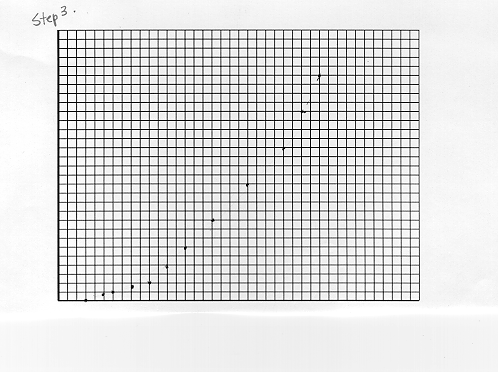+ Text Only Site
+ Non-Flash Version
+ Contact GlennStep 2.

Using the chart below, record the Airspeed and the Lift. By changing the Airspeed, make at least 9 additional readings. Record these in the chart.

Answers will vary. Samples are shown below:

 Airspeed (km/hr) Lift (Newtons) 0 0 38 401.5 56 919.8 95 2647.0 126 4437 171 8577 228 15,250 308 27,820 359 37,800 400 46,930

Step 3.

Enter your data into the Lists in your calculator. Set up Stat Plot so that the Airspeed is your independent variable and the Lift is the dependent variable. Graph the data on your calculator and make a sketch in the graph below.Step 4.

In FoilSim, push the Plots output button and select Lift vs. Speed. How does this graph compare to yours?

The graph should be very similar.

Step 5.

Which kind of function does this graph represent? The graph represents a parabola.

Step 6.

Using the regression equations (http://www.ti.com/calc/docs/act/pdf/marcus09.pdf) from your calculator, determine the equation of best fit.

a = .295
b = -.455
c = -8.901
R2 = .999
equation: .295X2 - .455X - 8.901

Step 7.

What does this equation tell you about the relationship between the change in Lift and the change in Airspeed? As the airspeed increases, the lift increases by more than the square of the airspeed.

Step 8.

Using the graph and/or the equation, what would you predict the lift to be given an airspeed of 300 km/hr.?

equation: .295(300)2 - .455 (300) -8.901
Lift = 26,404.59 Newtons
(Note: Have students check their answers using FoilSim: lift = 26,400 Newtons. Then take it one step farther and show the regression equation on a calculator.)

Related Pages:
Standards
Activity
Worksheet
Lesson Index
Aerodynamics Index+ Inspector General Hotline + Equal Employment Opportunity Data Posted Pursuant to the No Fear Act + Budgets, Strategic Plans and Accountability Reports + Freedom of Information Act + The President's Management Agenda + NASA Privacy Statement, Disclaimer, and Accessibility CertificationEditor: Tom Benson NASA Official: Tom Benson Last Updated: Thu, May 13 02:38:38 PM EDT 2021 + Contact Glenn